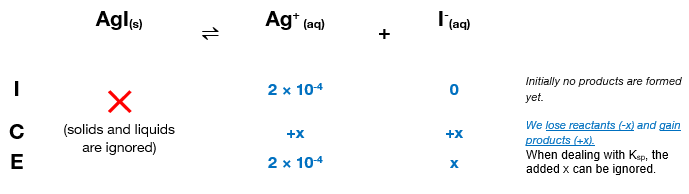# Problem: A solution contains 2.0 x 10-4 M Ag+ and 1.5 x 10-3 M Pb2+. If NaI is added, will AgI (Ksp = 8.3 x 10-17) or PbI2 (Ksp = 7.9 x 10-9) precipitate first? Specify the concentration of I- needed to begin precipitation.

###### FREE Expert Solution

Step 1: Calculate the I- needed to precipitate AgI and PbI2.

For AgI

Calculate the concentration of I needed in order to precipitate Ag+.

The compound is an ionic compound, it forms ions when dissociating in water. The dissociation of AgI is:

AgI(s)  Ag+(aq) + I(aq)

We can construct an ICE table for the dissociation of AgI. Remember that solids are ignored in the ICE table.The Ksp expression for AgI is:

Solids and liquids are ignored in the expression.

Note that each concentration is raised by the stoichiometric coefficient: both [Ag+] and [I] are raised to 1.

We can now plug in the equilibrium concentrations and solve for x, which is the molar concentration of I needed to start precipitation or the molar solubility of AgI.

x = 4.15×10-13 M = [I-]

88% (379 ratings)###### Problem Details

A solution contains 2.0 x 10-4 M Ag+ and 1.5 x 10-3 M Pb2+. If NaI is added, will AgI (Ksp = 8.3 x 10-17) or PbI2 (Ksp = 7.9 x 10-9) precipitate first? Specify the concentration of I- needed to begin precipitation.

Frequently Asked Questions

What scientific concept do you need to know in order to solve this problem?

Our tutors have indicated that to solve this problem you will need to apply the Ksp concept. You can view video lessons to learn Ksp. Or if you need more Ksp practice, you can also practice Ksp practice problems.

What professor is this problem relevant for?

Based on our data, we think this problem is relevant for Professor Underwood's class at FIU.